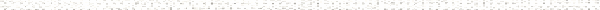An Engineering Statics Outline

 a. Representing Interactions Between BodiesObjective: Define interaction. Recognize attributes of interactions that can be represented by concentrated forces and the implications of Newton's 3rd law of motion.Objective: Identify attributes of forces acting between frictionless contacting bodies.Objective: Identify attributes of forces acting between a body and an attached cable.Objective: Identify attributes of forces acting between a body and an attached spring.Objective: Recognize that all the interactions between bodies that are in contact or attached to one another can be represented as vectors. Be able to draw force vectors on bodies to reflect their attributes. b. Effects of a ForceObjective: Distinguish between various motions produced by forces on bodiesObjective: Recognize qualitatively that the translational effects of a force depend on force magnitude, direction and sense, but do not depend on the point of application of the forceObjective: Recognize qualitatively that the rotational effects of a force depend on force magnitude, direction and sense, and on the line of action of the forceObjective: Define the tendency of the force to create rotation as the moment, and determine the moment of a force by identifying the perpendicular distance from the axis of rotation to the line of action of the force in 2D situationsObjective: Determine the moment of a force in 3D situations that can be modeled in 2D c. Effects of Multiple ForcesObjective: Recognize that translational effects of forces combine as in vector additionObjective: Determine the components of force vectorsObjective: Determine the sum of concurrent forces by summing their components.Objective: Recognize that rotational effects of forces combine through the addition of moments.Objective: Add moments due to known forces when their moment arms are given.Objective: Find the force that balances the moment created by several other forces when moment arms are given.Objective: Determine the moment of a force as a sum of the moments of its vertical and horizontal components.Objective: Determine the moment of a force as a sum of the moments of its components parallel and perpendicular to the line between the moment center and the point of application of the force.Objective: Judiciously choose method of calculating the moment of a forceObjective: Forces translated into a momentObjective: Shear and bending moment in beams d. Introduction to Free Body DiagramsObjective: Explain the purpose and philosophy of drawing FBDsObjective: Demonstrate the procedure of drawing FBDsObjective: Identify the factors which affect the choice of subsystem, and choose an appropriate subsystem in simple cases e. Equilibrium Under 2D Concentrated ForcesObjective: Recognize the equilibrium conditions under which bodies have no tendency to translate or rotateObjective: Impose the conditions of equilibrium when the equilibrium of moments on the body is trivially satisfied in systems with collinear forces for which the net moment about any point on the line is zeroObjective: Impose the conditions of equilibrium when the equilibrium of moments on the body is trivially satisfied in systems with concurrent forces for which the net moment about a point of intersection is zeroObjective: Impose the conditions of equilibrium when both force and moment equilibrium need to be explicitly satisfied in systems where all forces act in one directionObjective: Impose the conditions of equilibrium when both force and moment equilibrium need to be explicitly satisfied in systems with general coplanar forces